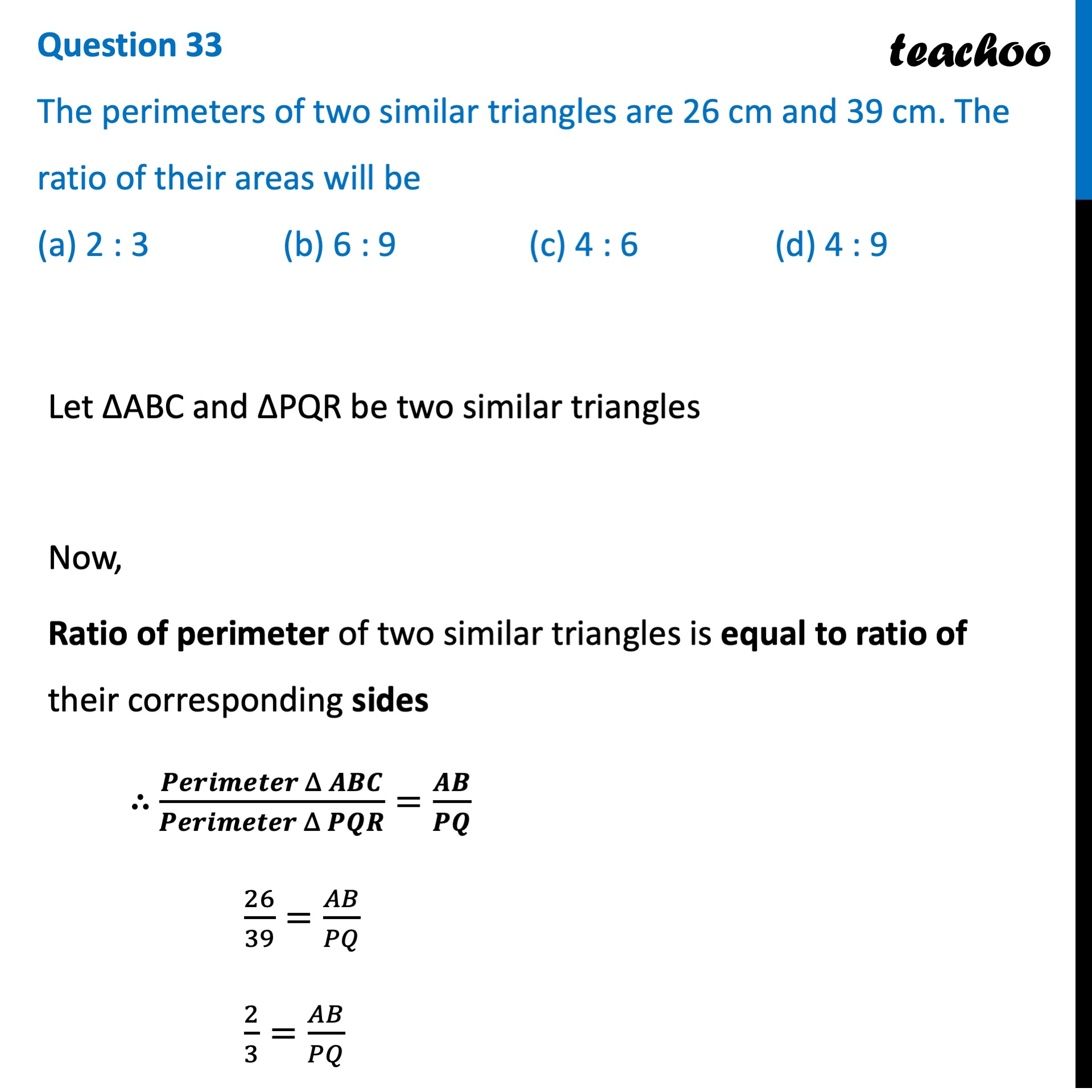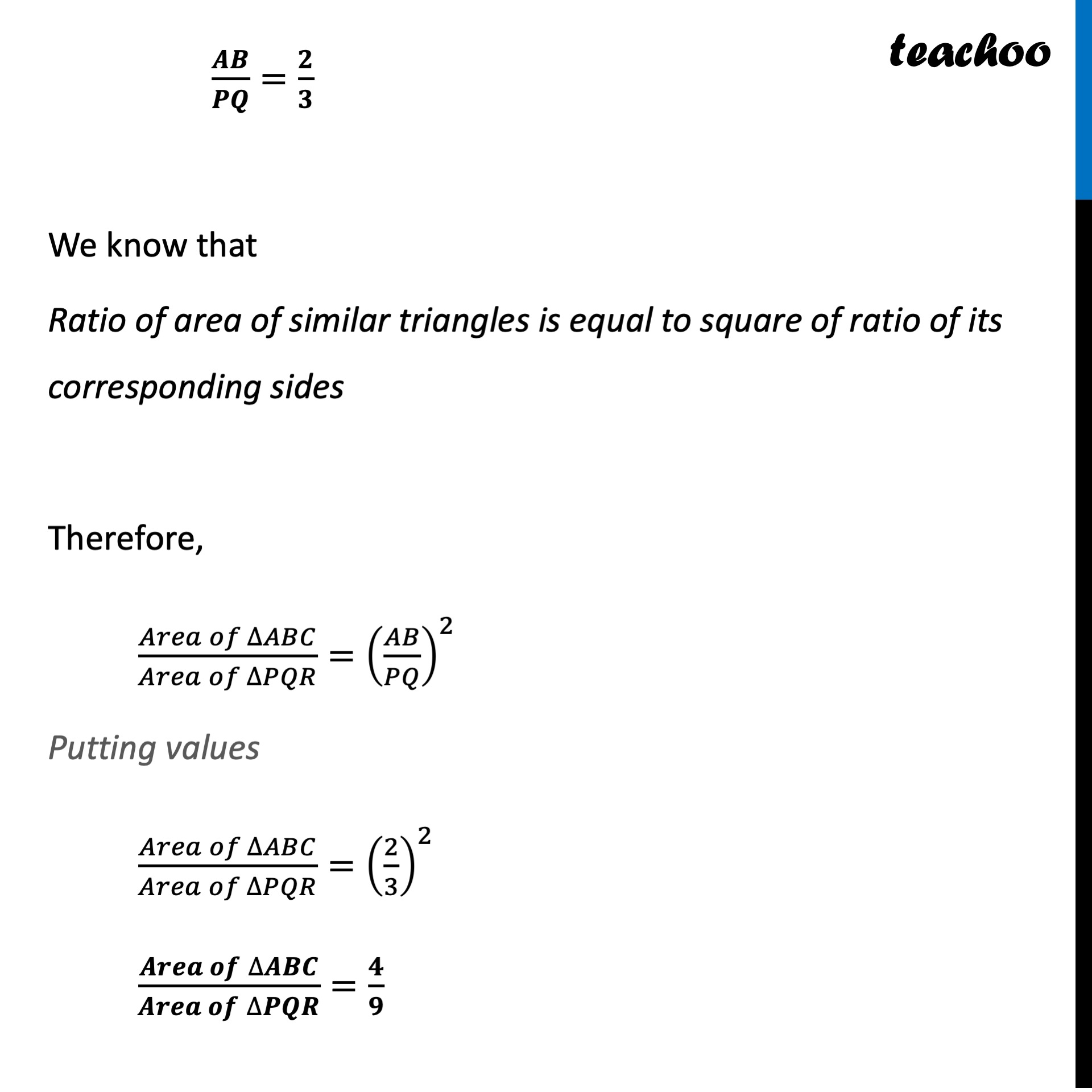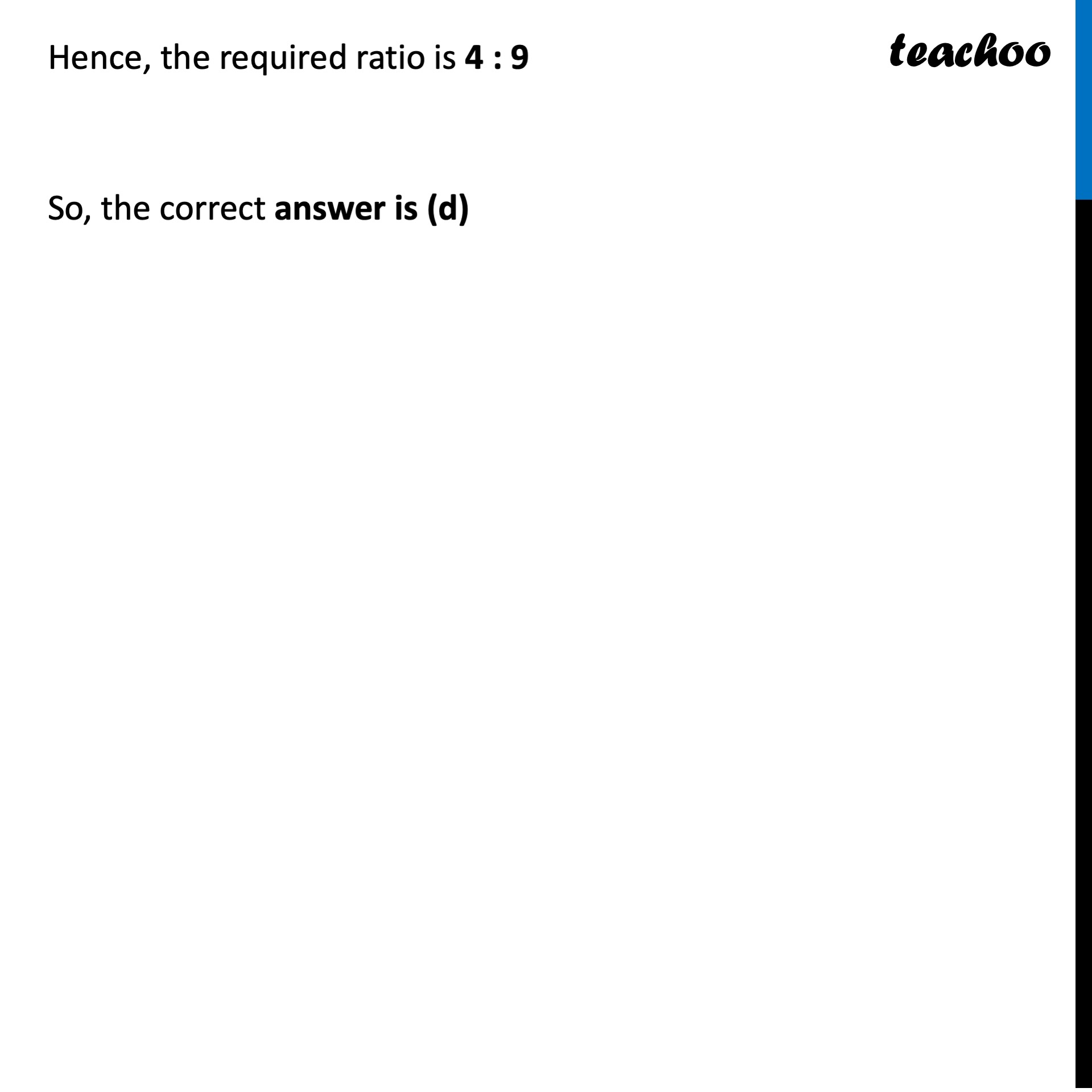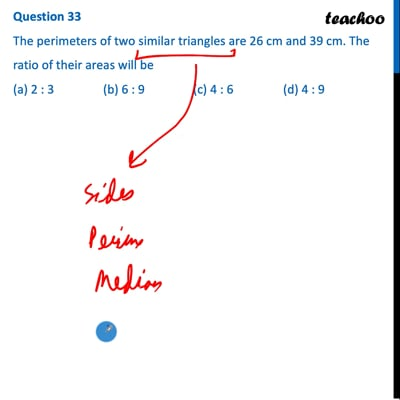CBSE Class 10 Sample Paper for 2022 Boards - Maths Basic [MCQ]

Class 10
Solutions of Sample Papers for Class 10 Boards

## (a) 2 : 3   (b) 6 : 9   (c) 4 : 6   (d) 4 : 9

This question is inspired from Question 13 - Sample Papers 2020 - Maths Standard - [Also, check proof of the formula]This video is only available for Teachoo black users

Learn in your speed, with individual attention - Teachoo Maths 1-on-1 Class

### Transcript

Question 33 The perimeters of two similar triangles are 26 cm and 39 cm. The ratio of their areas will be (a) 2 : 3 (b) 6 : 9 (c) 4 : 6 (d) 4 : 9 Let ΔABC and ΔPQR be two similar triangles Now, Ratio of perimeter of two similar triangles is equal to ratio of their corresponding sides ∴ (𝑷𝒆𝒓𝒊𝒎𝒆𝒕𝒆𝒓 Δ 𝑨𝑩𝑪)/(𝑷𝒆𝒓𝒊𝒎𝒆𝒕𝒆𝒓 Δ 𝑷𝑸𝑹)=𝑨𝑩/𝑷𝑸 26/39=𝐴𝐵/𝑃𝑄 2/3=𝐴𝐵/𝑃𝑄 𝑨𝑩/𝑷𝑸=𝟐/𝟑 We know that Ratio of area of similar triangles is equal to square of ratio of its corresponding sides Therefore, (𝐴𝑟𝑒𝑎 𝑜𝑓" " ∆𝐴𝐵𝐶)/(𝐴𝑟𝑒𝑎 𝑜𝑓" " ∆𝑃𝑄𝑅)=(𝐴𝐵/𝑃𝑄)^2 Putting values (𝐴𝑟𝑒𝑎 𝑜𝑓" " ∆𝐴𝐵𝐶)/(𝐴𝑟𝑒𝑎 𝑜𝑓" " ∆𝑃𝑄𝑅)=(2/3)^2 (𝑨𝒓𝒆𝒂 𝒐𝒇" " ∆𝑨𝑩𝑪)/(𝑨𝒓𝒆𝒂 𝒐𝒇" " ∆𝑷𝑸𝑹)=𝟒/𝟗 Hence, the required ratio is 4 : 9 So, the correct answer is (d)